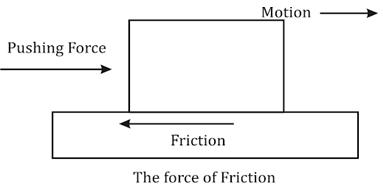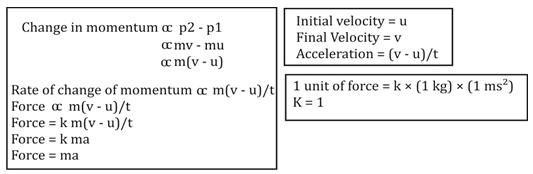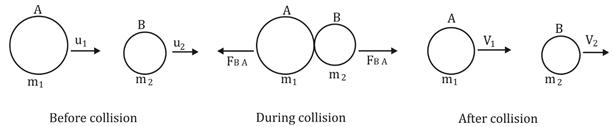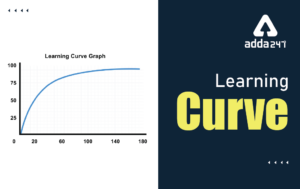Latest Teaching jobs   »   Force And Laws Of Motion: Science...

# Force And Laws Of Motion: Science Notes for CTET 2020: FREE PDF## FORCE AND LAWS OF MOTION

Force: Whenever we push or pull an object a force acts upon them and makes them move from one place to another.
Force can –
• Initiate motion in a motionless object
• Change (increase or decrease) the velocity of the moving object
• Alter the direction of a moving object
• Change the shape and size of an object

## Balanced and Unbalanced Forces

Balanced Forces:
• When equal amount of forces are applied on an object from different directions such that they cancel out each other
• They do not change the state of rest or motion of an object
• They may change the shape and size of an object

Unbalanced Force:
• When forces applied to an object are of different magnitude (or not in opposite directions so as to cancel)
• They can alter state of rest or motion of an object
• They can cause acceleration in an object
• They can change the shape and size of an object

What is the force of friction?
It is a force extended when two surfaces are in contact with each other. It always acts in a direction opposite to the direction of motion of the object.## Three Laws of Motion:

First law of motion or The Law of Inertia:
• Whether an object is moving uniformly on a straight path or is at rest, its state would not change until and unless an external force is applied on to it.
• Hence, we can say that objects oppose a change in their state of motion or rest. This tendency of objects to remain in the state of rest or to keep moving uniformly is called Inertia.

Examples of Inertia
• We fall back when a vehicle starts moving in the forward direction because our body is in the rest state and it opposes the motion of the vehicle.
• We fall forward when brakes are applied in a car because our body opposite the change of state of motion to rest

Inertia and Mass
• The inertia of an object is dependent upon its mass.
• Lighter objects have less inertia, that is, they can easily change their state of rest or motion.
• Heavier objects have large inertia and therefore they show more resistance.
• Hence ‘Mass’ is called a measure of the inertia of an object.

The Second Law of Motion:
The impact produced by a moving object depends upon its mass and velocity.
Example: a small bullet fired at a high velocity can kill a person.

Momentum – The product of mass and velocity is called Momentum.
It is a vector quantity. Its direction is same as that of the object’s velocity.
Denoted by – p
SI unit – kg metre per second
p = mv,
Where, m is the mass of the object,
v is the velocity of the object

The momentum of a stationary object –
Let the mass of a stationary object be ‘m’,
Let the velocity of a stationary object be ‘v’,
The stationary object has no velocity, so v = 0,
Therefore, p = m × v = m × 0 = 0
So, the momentum of a stationary object is zero.

The velocity of an object can be changed by applying an unbalanced force on to it. Similarly, the momentum of an object can change by applying an unbalanced force.

According to the second law of motion –
The rate of change of momentum of an object is directly proportional to the applied unbalanced force on the object in the direction of the force.
Example
• A cricketer when catches a ball pulls his hands in the backward direction to give some time to decrease the velocity of the ball. As the acceleration of the ball decreases the force exerted on catching the moving ball also decreases. If the cricketer would try to stop a moving ball suddenly he would have to apply larger force.

Mathematical Formulation of the Second Law of Motion:
Based on the definition of the second law of motion, we can infer that –From the formula stated above, we can see that the force is directly proportional to acceleration. So the acceleration of an object can change depending upon the change in force applied.
Force = ma
SI Unit: kg-ms-2 or N (Newton)

The Third Law of Motion:

Action and Reaction Forces
Two forces acting from opposite directions are called Action and Reaction Forces.

Example, a ball when hits the ground (action) bounces back with a certain force reaction.

The Third Law of Motion States that –
When an object exerts a force on another object, the second object instantly exerts a force back onto the first object. These forces are always equal in magnitude but opposite in direction. These forces act on two different objects always.
Or in other words, every action has an equal and opposite reaction.
The magnitudes of forces acting upon the objects are same but the acceleration produced in them may or may not be the same because the objects can differ in masses.

Example, when a bullet is fired from a gun, the gun only moves a little backwards (recoils) while the bullet can travel a large distance. This is because of the difference in the mass of the bullet and the gun.

Conservation of Momentum:
The sum of momenta of two objects before the collision and after collision remains the same given that no external unbalanced force acts upon them. In another way, collision conserves the total momentum of two objects.Consider the figure given above. Two balls A and B having a certain initial velocities collide with each other. Conditions before the collision-
• There is no unbalanced force acting upon them
• The initial velocity of A is greater than initial velocity of B

• They are considered as the fundamental laws in physics.
• They are based on observations and experiments.
• They cannot be proved but can be verified or disproved with the help of experiments.
• A single experiment is enough to disprove a law, while a single experiment is not enough to prove the same.
• It requires a large number of experiments to prove the law.
• The law of conservation of momentum was formulated 300 years ago.
• There is no single situation present until now that disproves this law.

•Learning Curve - What is Learning Curve?...
•रस - परिभाषा, भे�...
•पठन कौशल- परिभा�...
•अलंकार - परिभाष�...
•Indian States and Capitals 2022: 28 Stat...
•New Education Policy(NEP) PDF - नई �...